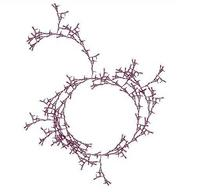University of Virginia, Department of Computer Science CS200: Computer Science, Spring 2003

Notes: Wednesday 25 March 2003

• Monday, 31 March: Problem Set 8 team requests (see Notes 21 for details)
• Friday, 4 April: Problem Set 7. Lab hours: Wednesday, 26 March: 6-7:30pm (Rachel); Thursday, 27 March: 7-8:30pm (Rachel); Tuesday, 1 April: 8-9:30pm (Jacques); Wednesday, 2 April: 8-9:30pm (Jacques)

Meta-Circular Evaluator
```(define (meval expr env)
(cond
((self-evaluating? expr) expr)
((quoted? expr)          (text-of-quotation expr))
((definition? expr)      (define-variable!
(definition-variable expr)
(meval (definition-value expr) env) env))
((lambda? expr)          (make-procedure (lambda-parameters expr) (lambda-body expr) env))
((application? expr)     (mapply (meval (application-operator expr) env)
(map (lambda (subexpr) (meval subexpr env))
(application-operands expr))))
((variable? expr)        (environment-lookup-name expr env)) ;; Must be last
(else (error "Unrecognized expression: " expr))))

(define (mapply procedure operands)
(cond
((primitive-procedure? procedure) (apply-primitive procedure operands))
((compound-procedure? procedure)  (apply-compound procedure operands))
(else (error "Unknown applicator: " procedure))))

(define (apply-primitive procedure operands)
;;; Uses the underlying Scheme apply
(apply (primitive-procedure-procedure procedure) operands))

(define (apply-compound procedure operands)
(meval-sequence
(procedure-body procedure)
(extend-environment (procedure-parameters procedure)
operands
(procedure-environment procedure))))

(define (meval-sequence seq env)
;;; Evaluate to the value of the last expression in the sequence
(get-last (map (lambda (expr) (meval expr env)) seq)))
```
```;;;
;;; Environments
;;;

(define the-empty-environment '())

(define (make-new-environment frame env) (cons frame env))
(define (make-empty-frame) (list))

(define (first-frame env) (car env))
(define (enclosing-environment env) (cdr env))

(define (extend-environment names values env)
(make-new-environment
(map (lambda (name value) (cons name value)) names values)
env))

(define (environment-lookup-name name env)
(if (null? env) (error "No binding for" name)
(if (frame-contains? name (first-frame env))
(frame-lookup-name name (first-frame env))
(environment-lookup-name name (enclosing-environment env)))))

(define (bind-variable name value env)

(car frame) ;; ! frame cannot be null or append! doesn't work
(append! frame (list (cons name value)))) ; Note why we use append! not append

(define (frame-contains? name frame)
(insertl
(lambda (var result) (if (eq? (car var) name) #t result))
frame
#f))

(define (frame-lookup-name name frame)
(if (eq? (car (car frame)) name)
(cdr (car frame))
(frame-lookup-name name (cdr frame)))))

(define (define-variable! var value env)
;;; We always add the new definition at the beginning of the frame.  That way,
;;; frame-lookup-name will find the new definition first if it is redefined.
(set-car! env (cons (cons var value) (car env))))

;;; Mini-scheme can add, multiply and subtract using the underlying Scheme primitives:

(define the-global-environment
(make-new-environment
(list
;; Some popular constants
(cons 'true #t)
(cons 'false #f)
(cons 'null null)
;; Primitive procedures
(cons '+ (make-primitive-procedure +))
(cons '* (make-primitive-procedure *))
(cons '- (make-primitive-procedure -))
;;; ... some more in PS7's meval.ss
)
the-empty-environment))
```
```;;; We represent different entities using tagged lists.

(define (tagged-list? expr tag)
(if (pair? expr) (eq? (car expr) tag) #f))

(define (self-evaluating? expr)
(or (number? expr) (string? expr) (primitive-procedure? expr)))

(define (variable? expr) (symbol? expr))

(define (quoted? expr) (tagged-list? expr `quote))

(define (definition? expr) (tagged-list? expr 'define))

(define (lambda? expr) (tagged-list? expr 'lambda))
(define (make-lambda params body) (list 'lambda params body))
(define (lambda-body expr) (cddr expr))

(define (and? expr) (tagged-list? expr 'and))
(define (make-and operands) (list 'and operands))
(define (and-operands expr) (cdr expr))

(define (make-procedure parameters body environment)
(list 'procedure parameters body environment))

(define (compound-procedure? expr) (tagged-list? expr 'procedure))CS 200: Computer Science Department of Computer Science University of Virginia Circle Fractal by Ramsey Arnaoot and Qi Wang cs200-staff@cs.virginia.edu Using these Materials